# Volumetric wavelet Data Processing¶

Important: Please read the installation page for details about how to install the toolboxes. $\newcommand{\dotp}{\langle #1, #2 \rangle}$ $\newcommand{\enscond}{\lbrace #1, #2 \rbrace}$ $\newcommand{\pd}{ \frac{ \partial #1}{\partial #2} }$ $\newcommand{\umin}{\underset{#1}{\min}\;}$ $\newcommand{\umax}{\underset{#1}{\max}\;}$ $\newcommand{\umin}{\underset{#1}{\min}\;}$ $\newcommand{\uargmin}{\underset{#1}{argmin}\;}$ $\newcommand{\norm}{\|#1\|}$ $\newcommand{\abs}{\left|#1\right|}$ $\newcommand{\choice}{ \left\{ \begin{array}{l} #1 \end{array} \right. }$ $\newcommand{\pa}{\left(#1\right)}$ $\newcommand{\diag}{{diag}\left( #1 \right)}$ $\newcommand{\qandq}{\quad\text{and}\quad}$ $\newcommand{\qwhereq}{\quad\text{where}\quad}$ $\newcommand{\qifq}{ \quad \text{if} \quad }$ $\newcommand{\qarrq}{ \quad \Longrightarrow \quad }$ $\newcommand{\ZZ}{\mathbb{Z}}$ $\newcommand{\CC}{\mathbb{C}}$ $\newcommand{\RR}{\mathbb{R}}$ $\newcommand{\EE}{\mathbb{E}}$ $\newcommand{\Zz}{\mathcal{Z}}$ $\newcommand{\Ww}{\mathcal{W}}$ $\newcommand{\Vv}{\mathcal{V}}$ $\newcommand{\Nn}{\mathcal{N}}$ $\newcommand{\NN}{\mathcal{N}}$ $\newcommand{\Hh}{\mathcal{H}}$ $\newcommand{\Bb}{\mathcal{B}}$ $\newcommand{\Ee}{\mathcal{E}}$ $\newcommand{\Cc}{\mathcal{C}}$ $\newcommand{\Gg}{\mathcal{G}}$ $\newcommand{\Ss}{\mathcal{S}}$ $\newcommand{\Pp}{\mathcal{P}}$ $\newcommand{\Ff}{\mathcal{F}}$ $\newcommand{\Xx}{\mathcal{X}}$ $\newcommand{\Mm}{\mathcal{M}}$ $\newcommand{\Ii}{\mathcal{I}}$ $\newcommand{\Dd}{\mathcal{D}}$ $\newcommand{\Ll}{\mathcal{L}}$ $\newcommand{\Tt}{\mathcal{T}}$ $\newcommand{\si}{\sigma}$ $\newcommand{\al}{\alpha}$ $\newcommand{\la}{\lambda}$ $\newcommand{\ga}{\gamma}$ $\newcommand{\Ga}{\Gamma}$ $\newcommand{\La}{\Lambda}$ $\newcommand{\si}{\sigma}$ $\newcommand{\Si}{\Sigma}$ $\newcommand{\be}{\beta}$ $\newcommand{\de}{\delta}$ $\newcommand{\De}{\Delta}$ $\newcommand{\phi}{\varphi}$ $\newcommand{\th}{\theta}$ $\newcommand{\om}{\omega}$ $\newcommand{\Om}{\Omega}$

This numerical tour explores volumetric (3D) data processing.

In :
using PyPlot
using NtToolBox
arequire("NtToolBox")

WARNING: Method definition macroexpand(Module, Any) in module Compat at C:\Users\Ayman\.julia\v0.5\Compat\src\Compat.jl:1491 overwritten in module MacroTools at C:\Users\Ayman\.julia\v0.5\MacroTools\src\utils.jl:64.
WARNING: Base.UTF8String is deprecated, use String instead.
WARNING: Base.UTF8String is deprecated, use String instead.
WARNING: Base.UTF8String is deprecated, use String instead.
WARNING: Base.UTF8String is deprecated, use String instead.
WARNING: Base.UTF8String is deprecated, use String instead.
WARNING: Base.UTF8String is deprecated, use String instead.
likely near In:4
WARNING: Base.UTF8String is deprecated, use String instead.
likely near In:4
WARNING: Base.UTF8String is deprecated, use String instead.
likely near In:4
WARNING: Base.UTF8String is deprecated, use String instead.
likely near In:4
WARNING: Base.UTF8String is deprecated, use String instead.
likely near In:4
WARNING: Base.UTF8String is deprecated, use String instead.
likely near In:4
WARNING: Base.UTF8String is deprecated, use String instead.
likely near In:4
WARNING: Base.UTF8String is deprecated, use String instead.
likely near In:4
WARNING: Base.UTF8String is deprecated, use String instead.
likely near In:4
WARNING: Base.UTF8String is deprecated, use String instead.
likely near In:4
WARNING: Base.UTF8String is deprecated, use String instead.
likely near In:4
WARNING: Base.UTF8String is deprecated, use String instead.
likely near In:4
WARNING: Base.UTF8String is deprecated, use String instead.
likely near In:4
WARNING: Base.UTF8String is deprecated, use String instead.
likely near In:4
WARNING: Base.UTF8String is deprecated, use String instead.
likely near In:4
WARNING: Base.UTF8String is deprecated, use String instead.
likely near In:4
WARNING: Base.UTF8String is deprecated, use String instead.
likely near In:4
WARNING: Base.UTF8String is deprecated, use String instead.
likely near In:4
WARNING: Base.UTF8String is deprecated, use String instead.
likely near In:4
WARNING: Base.UTF8String is deprecated, use String instead.
likely near In:4
WARNING: Base.UTF8String is deprecated, use String instead.
likely near In:4
WARNING: Base.UTF8String is deprecated, use String instead.
likely near In:4
WARNING: Base.UTF8String is deprecated, use String instead.
likely near In:4
WARNING: Base.UTF8String is deprecated, use String instead.
likely near In:4
WARNING: Base.UTF8String is deprecated, use String instead.
likely near In:4
WARNING: Base.UTF8String is deprecated, use String instead.
likely near In:4
WARNING: Base.UTF8String is deprecated, use String instead.
likely near In:4
WARNING: Base.UTF8String is deprecated, use String instead.
likely near In:4
WARNING: Base.UTF8String is deprecated, use String instead.
likely near In:4
WARNING: Base.UTF8String is deprecated, use String instead.
likely near In:4
WARNING: Base.UTF8String is deprecated, use String instead.
likely near In:4
WARNING: Base.UTF8String is deprecated, use String instead.
likely near In:4
WARNING: Base.UTF8String is deprecated, use String instead.
likely near In:4
WARNING: Base.UTF8String is deprecated, use String instead.
likely near In:4
WARNING: Base.UTF8String is deprecated, use String instead.
likely near In:4
WARNING: Base.UTF8String is deprecated, use String instead.
likely near In:4
WARNING: Base.UTF8String is deprecated, use String instead.
likely near In:4
WARNING: Base.UTF8String is deprecated, use String instead.
likely near In:4
WARNING: Base.UTF8String is deprecated, use String instead.
likely near In:4
WARNING: Base.UTF8String is deprecated, use String instead.
likely near In:4
in depwarn(::String, ::Symbol) at .\deprecated.jl:64
in #parse_file#6(::Array{Any,1}, ::Function, ::String) at C:\Users\Ayman\.julia\v0.5\Autoreload\src\files.jl:63
in execute_request(::ZMQ.Socket, ::IJulia.Msg) at C:\Users\Ayman\.julia\v0.5\IJulia\src\execute_request.jl:157
in eventloop(::ZMQ.Socket) at C:\Users\Ayman\.julia\v0.5\IJulia\src\eventloop.jl:8
WARNING: replacing module NtToolBox
WARNING: Base.UTF8String is deprecated, use String instead.
likely near In:5
WARNING: Base.UTF8String is deprecated, use String instead.
likely near In:5
WARNING: Base.UTF8String is deprecated, use String instead.
likely near In:5
WARNING: Base.UTF8String is deprecated, use String instead.
likely near In:5
WARNING: Base.UTF8String is deprecated, use String instead.
likely near In:5
WARNING: Base.UTF8String is deprecated, use String instead.
likely near In:5


## 3D Volumetric Datasets¶

In :
M = NtToolBox.read_bin("NtToolBox/src/data/vessels.bin", 3);

In :
M = NtToolBox.rescale(M);


Size of the image (here it is a cube).

In :
n = size(M);


We can display some horizontal slices.

In :
slices = Array{Int64,1}(round(linspace(10, n-10, 4)))
figure(figsize = (10,10))

for i in 1:length(slices)
s = slices[i]
NtToolBox.imageplot(M[:, :, s], @sprintf("Z = %i", s), [2, 2, i])
end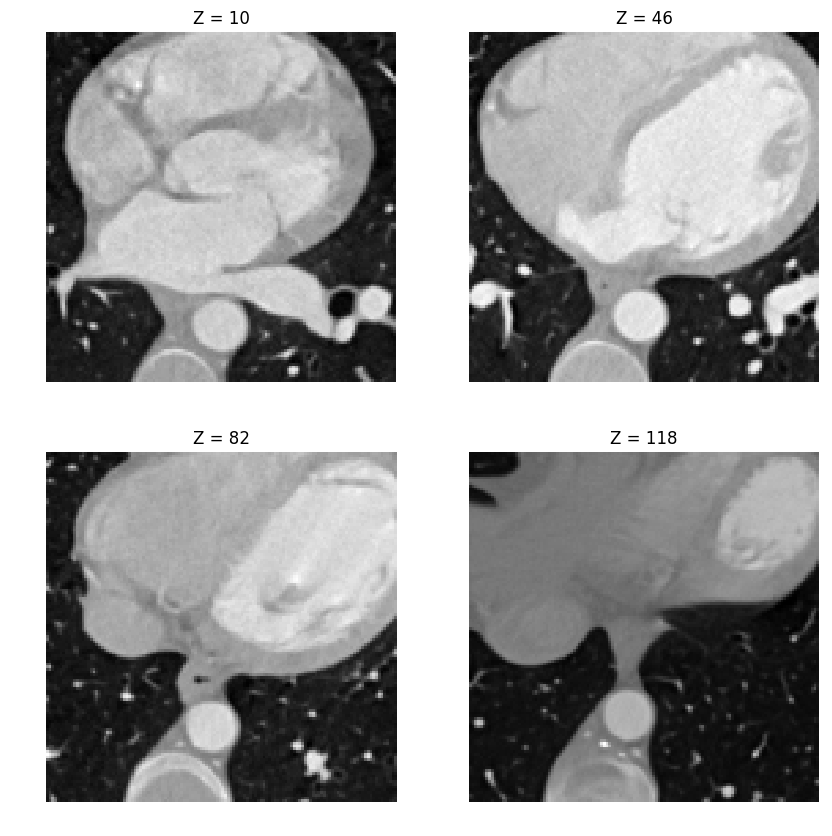We can display an isosurface of the dataset (here we sub-sample to speed up the computation). You need to have PyCall package installed in Julia in order to use some functions in python, besides you also have to install the package skimage in python.

In :
include("NtToolBox/src/isosurface.jl")
isosurface(M, .5, 3, "")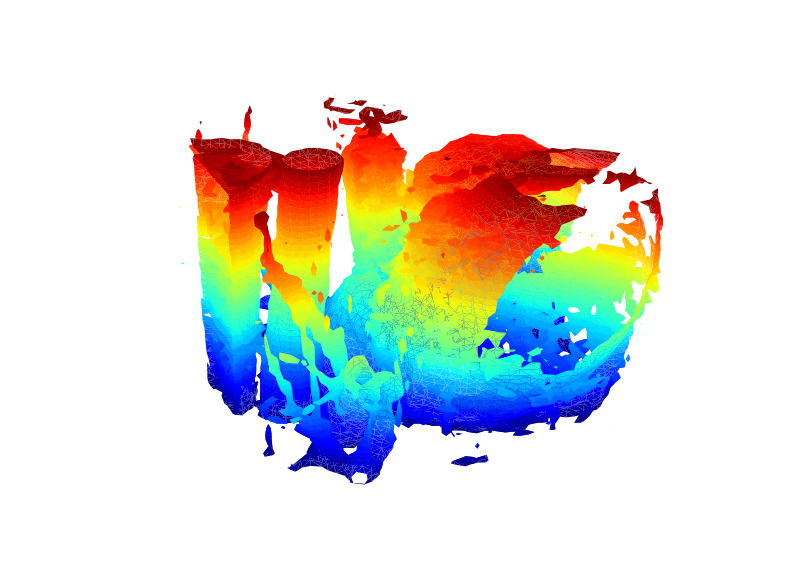## 3D Haar Transform¶

An isotropic 3D Haar transform recursively extracts details wavelet coefficients by performing local averages/differences along the X/Y/Z axis.

We apply a step of Haar transform in the X/Y/Z direction

Initialize the transform

In :
MW = copy(M);


Average/difference along X

In :
MW = cat(1, (MW[1:2:n, :, :] + MW[2:2:n, :, :])./sqrt(2), (MW[1:2:n, :, :] - MW[2:2:n, :, :])./sqrt(2) );


Average/difference along Y

In :
MW = cat(2, (MW[:, 1:2:n, :] + MW[:, 2:2:n, :])./sqrt(2), (MW[:, 1:2:n, :] - MW[:, 2:2:n, :])./sqrt(2) );


Average/difference along Z

In :
MW = cat(3, (MW[:, :, 1:2:n] + MW[:, :, 2:2:n])./sqrt(2), (MW[:, :, 1:2:n] - MW[:, :, 2:2:n])./sqrt(2) );


Display a horizontal and vertical slice to see the structure of the coefficients.

In :
figure(figsize = (10, 5))
imageplot(MW[:, :, 30], "Horizontal slice", [1,2,1])
imageplot((MW[:, 30, :]), "Vertical slice", [1,2,2])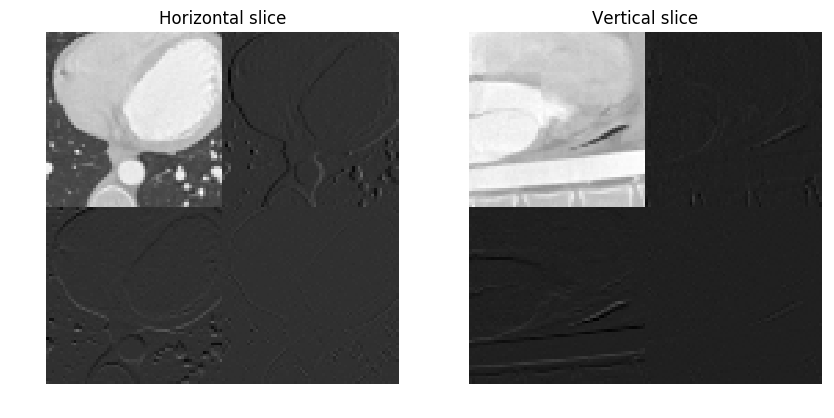Out:
PyObject <matplotlib.text.Text object at 0x000000002F2CD208>

Exercise 1

Implement the forward wavelet transform by iteratively applying these transform steps to the low pass residual.

In :
include("NtSolutions/multidim_2_volumetric/exo1.jl")

In :
## Insert your code here.


## Volumetric Data Haar Approximation¶

An approximation is obtained by keeping only the largest coefficients.

We threshold the coefficients to perform $m$-term approximation.

number of kept coefficients

In :
m = Int(round(.01*n^3))
MWT = NtToolBox.perform_thresholding(MW, m, "largest");


Exercise 2

Implement the backward transform to compute an approximation $M_1$ from the coefficients MWT.

In :
include("NtSolutions/multidim_2_volumetric/exo2.jl")

In :
## Insert your code here.


Display the approximation as slices.

In :
s = 30

figure(figsize = (10, 5))
imageplot(M[:, :, s], "Original", [1, 2, 1])
imageplot(clamP(M1[:, :, s]), "Approximation", [1,2,2])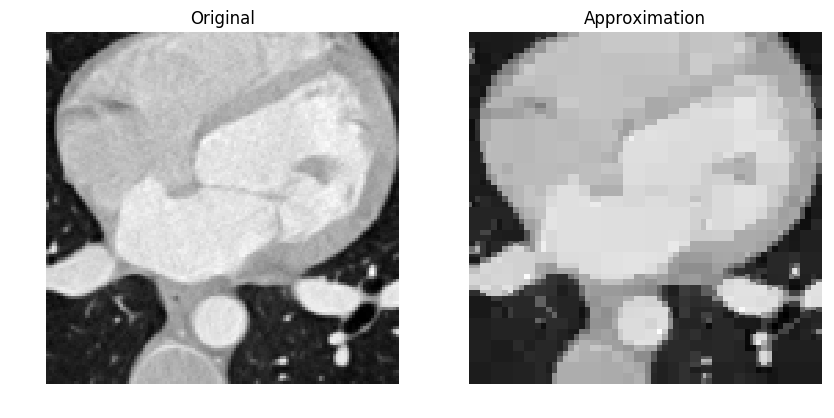Out:
PyObject <matplotlib.text.Text object at 0x000000002F36B160>

Display the approximated isosurface.

In :
isosurface(M1, .5, 2, "")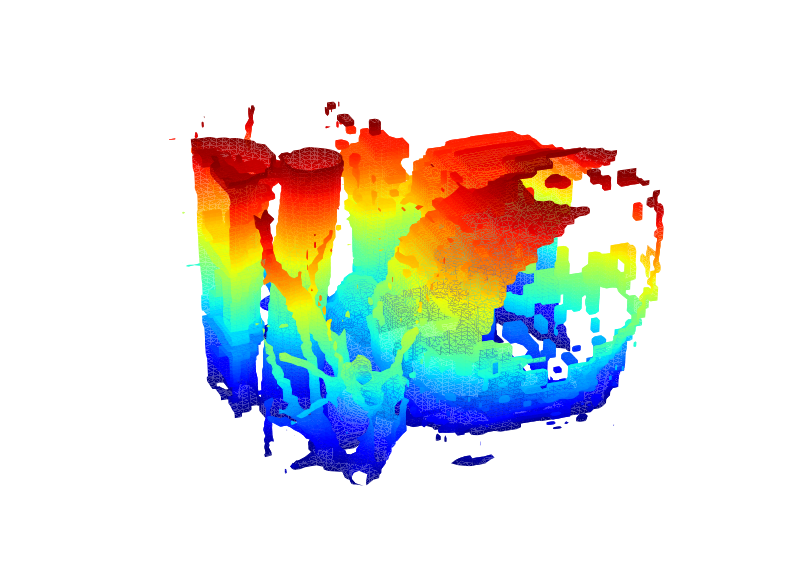## Linear Volumetric Denoising ¶

Linear denoising is obtained by low pass filtering.

We add a Gaussian noise to the image.

In :
sigma = .06
Mnoisy = M + sigma.*randn(n, n, n);


Display slices of the noisy data.

In :
figure(figsize = (10, 5))
imageplot(Mnoisy[:, :, Base.div(n, 2)], "X slice", [1,2,1])
imageplot(Mnoisy[:, Base.div(n, 2), :], "Y slice", [1,2,2])